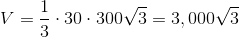# ISEE Upper Level Math : How to find the volume of a tetrahedron

## Example Questions

### Example Question #4 : Tetrahedrons

In three-dimensional space, the four vertices of a tetrahedron - a solid with four faces - have Cartesian coordinates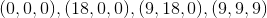.

Give its volume.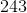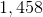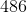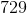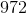Explanation:

A tetrahedron is a triangular pyramid and can be looked at as such.

Three of the vertices -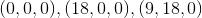- are on the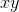-plane, and can be seen as the vertices of the triangular base. This triangle, as seen below, is isosceles: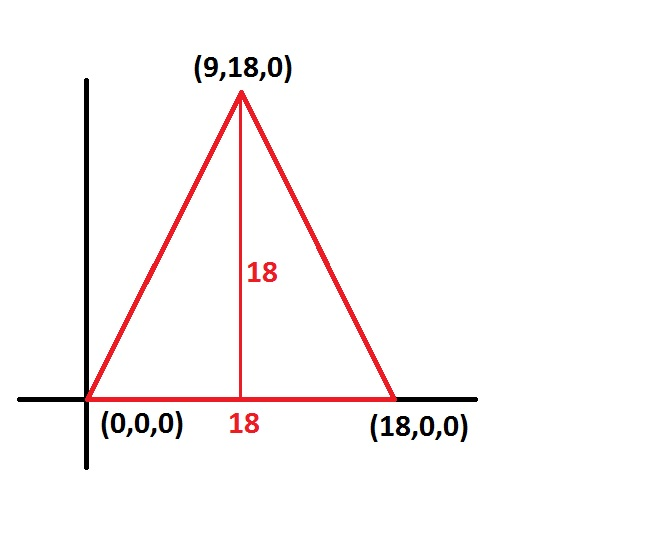Its base and height are both 18, so its area is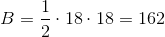The fourth vertex is off the-plane; its perpendicular distance to the aforementioned face is its-coordinate, 9, so this is the height of the pyramid. The volume of the pyramid is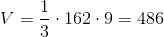### Example Question #5 : Tetrahedrons

In three-dimensional space, the four vertices of a tetrahedron - a solid with four faces - have Cartesian coordinates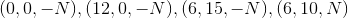, where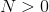.

Give its volume in terms of.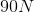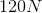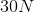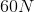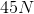Explanation:

A tetrahedron is a triangular pyramid and can be looked at as such.

Three of the vertices -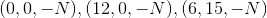- are on the horizontal plane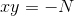, and can be seen as the vertices of the triangular base. This triangle, as seen below, is isosceles: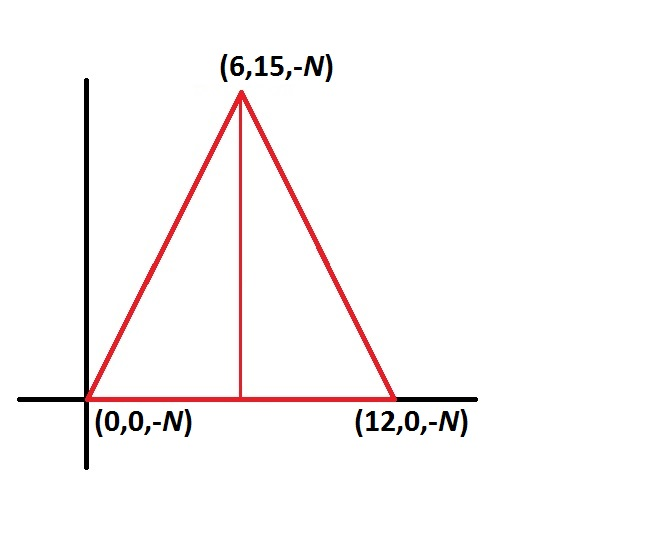Its base is 12 and its height is 15, so its area is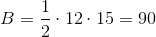The fourth vertex is off this plane; its perpendicular (vertical) distance to the aforementioned face is the difference between the-coordinates,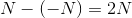, so this is the height of the pyramid. The volume of the pyramid is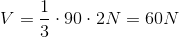### Example Question #1 : Tetrahedrons

In three-dimensional space, the four vertices of a tetrahedron - a solid with four faces - have Cartesian coordinates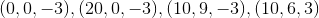.

Give its volume in terms of.The correct answer is not among the other choices.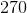Explanation:

A tetrahedron is a triangular pyramid and can be looked at as such.

Three of the vertices -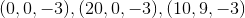- are on the horizontal plane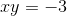, and can be seen as the vertices of the triangular base. This triangle, as seen below, is isosceles (drawing not to scale):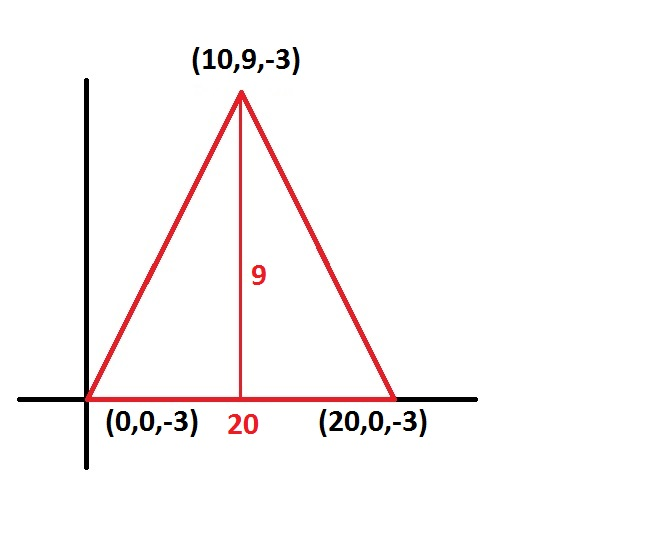Its base is 20 and its height is 9, so its area is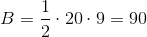The fourth vertex is off this plane; its perpendicular (vertical) distance to the aforementioned face is the difference between the-coordinates,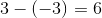, so this is the height of the pyramid. The volume of the pyramid is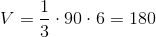### Example Question #7 : Tetrahedrons

In three-dimensional space, the four vertices of a tetrahedron - a solid with four faces - have Cartesian coordinates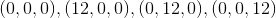.

What is the volume of this tetrahedron?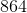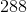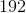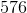Explanation:

The tetrahedron looks like this: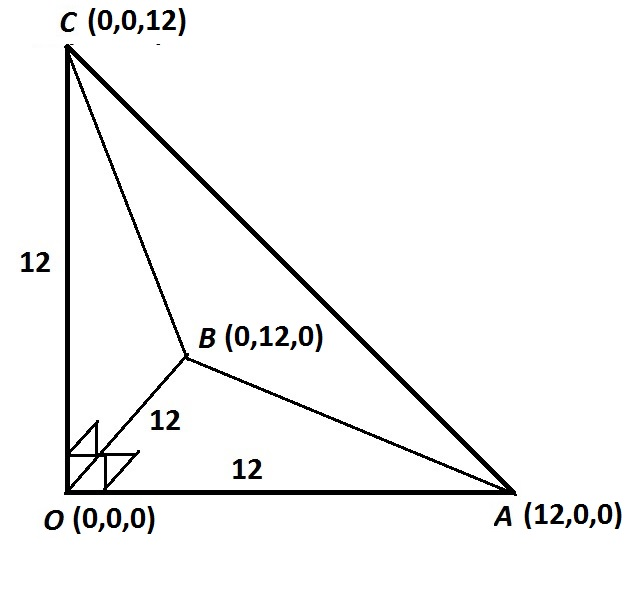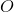is the origin and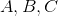are the other three points, which are twelve units away from the origin, each on one of the three (mutually perpendicular) axes.

This is a triangular pyramid, so look at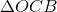as its base; the areaof the base is half the product of its legs, or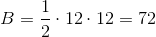.

The volume of the tetrahedron, it being essentially a pyramid, is one third the product of its base and its height, the latter of which is 12. Therefore,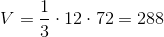.

### Example Question #8 : Tetrahedrons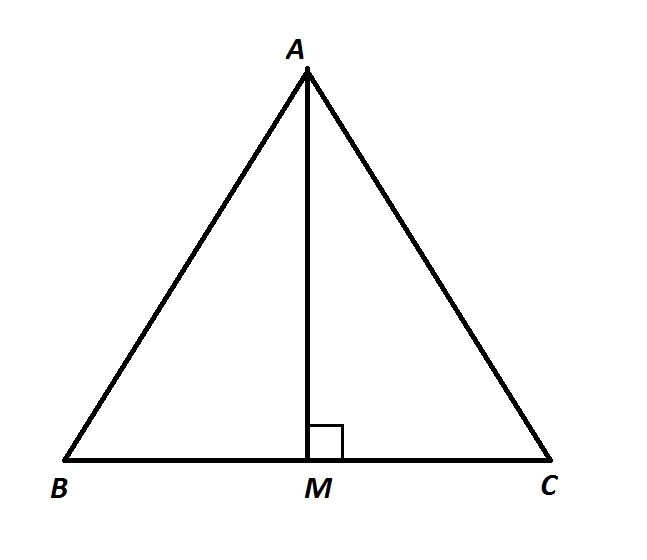Above is the base of a triangular pyramid, which is equilateral.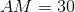, and the pyramid has height 30. What is the volume of the pyramid?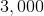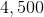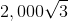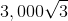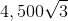Explanation:

Altitude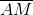divides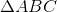into two 30-60-90 triangles.

By the 30-60-90 Theorem,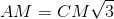, or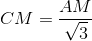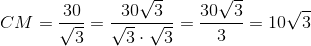is the midpoint of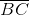, so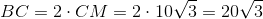The area of the triangular base is half the product of its base and its height: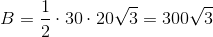The volume of the pyramid is one third the product of this area and the height of the pyramid: